# Quiz 1: Management Science

Business

A. The time taken by a bus to complete one round of the trip is 1.5 hours. There are 3 passenger buses available. Each bus makes 4 trips in a day. Total number of hours spent by 3 buses in making 4 trips each day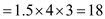hours. Operating cost per hour for each bus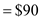Cost of operating 3 buses in a day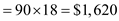Total cost of operating 3 buses for 365 days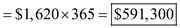Cost of purchasing a bus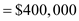Total cost of purchasing 3 buses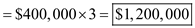Total cost of purchasing and operating the buses for 365 days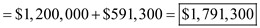Estimated number of passengers to travel in the bus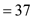Planned fare per rider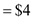Number of buses available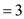Number of trips made by each bus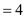Revenue earned by 3 buses that make 4 trips per day and carry an estimated 37 passengers with \$4 fare per rider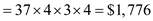Total revenue earned in 365 days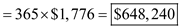Thus, the town will lose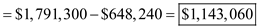B. Revenue earned by 3 buses in 365 days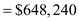Operating cost of 3 buses for 365 days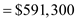Amount of money earned over the operating cost in 365 days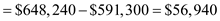Fixed cost spent in purchasing 3 buses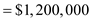Amount of time required to pay for the cost of 3 buses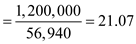Thus, a total of 21 year would be required for the town to break even and the town will not be able to break even during the life span of 6 years of the buses. C. In case the bus ridership averages 45 passengers, the revenue earned will beTotal revenue earned in 365 days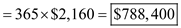Amount of money earned over the operating cost in 365 days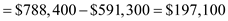Fixed cost spent in purchasing 3 busesAmount of time required to pay for the cost of 3 buses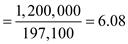Thus, the town would be able to break even in 6.08 years in case the ridership averages 45 passengers. In case the bus ridership averages 50 passengers, the revenue earned will be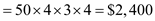Total revenue earned in 365 days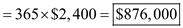Amount of money earned over the operating cost in 365 days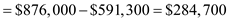Fixed cost spent in purchasing 3 busesAmount of time required to pay for the cost of 3 buses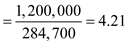Thus, the town would be able to break even in 4.21 years in case the ridership averages 50 passengers. D. The time taken by a bus to complete one round of the trip is 1.5 hours. There are 3 passenger buses available. Each bus makes 3 trips in a day. Total number of hours spent by 3 buses in making 3 trips each day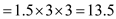hours. Operating cost per hour for each busCost of operating 3 buses in a day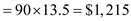Total cost of operating 3 buses for 365 days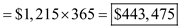Cost of purchasing a busTotal cost of purchasing 3 busesTotal cost of purchasing and operating the buses for 365 days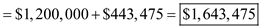Estimated number of passengers to travel in the bus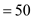Planned fare per riderNumber of buses availableNumber of trips made by each busRevenue earned by 3 buses that make 3 trips per day and carry an estimated 50 passengers with \$4 fare per rider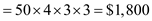Total revenue earned in 365 days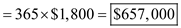Amount of money earned over the operating cost in 365 days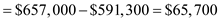Fixed cost spent in purchasing 3 busesAmount of time required to pay for the cost of 3 buses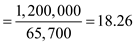Thus, the town would be able to break even in 18.26 years in case the ridership averages 50 passengers and the number of trips per bus is 3. E. In case the town is able to get federal grant to pay for the buses, then the fixed cost spent in purchasing the buses will be eliminated. Thus, the town will be no longer required to pay for the fixed cost which amounts to \$1,200,000. Thus, in the first year revenue earned is \$648,240 and the town would be required to pay only for the operating cost which is \$591,300. Hence, the town will earn a profit of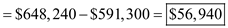The town would be able to break even in the first year itself.

a) The longer chamber of commerce is investing \$4.5 million to construct a parking lot for the town people. To pay for the project, they are selling municipal bonds with duration of 30 years at 8% interest. The annual cost for labor is \$140,000. The fee per car is \$3.20 and the variable cost is \$0.60. Determine the number of cars that would have to park in the lot on an annual basis in the 30-year time frame using the following break-even formula: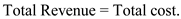…… (1) Calculate the total revenue and total cost as shown below: Consider the break-even sales as x.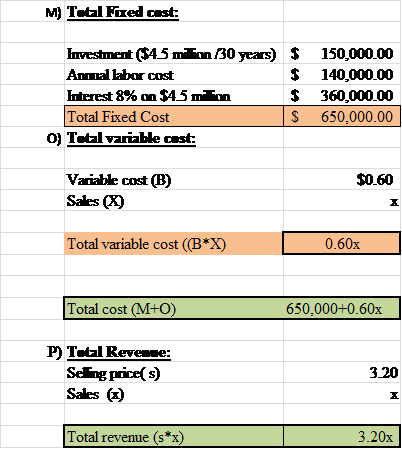Thus, the total revenue is 3.20 x and the total cost is 650,000 + 0.60x. Substitute the values in the equation …… (1) and calculate the number of cars to be parked in the lot as shown below: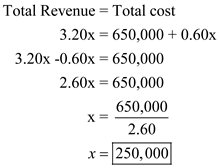Thus, in a year they should at least park 250,000 cars to pay off the project in 30-year time frame. b) Per year the total cars that should be parked is 250,000. Calculate for a day as shown below: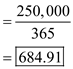Thus, per day they need 685 cars to be parked. It is feasible as the total population is 47,000. Per day 685 cars can be effortlessly parked.

The problem that one has before them here is to determine the total costs, revenues, and profits or losses that are applicable to the information presented for the Willow Furniture Company. A secondary part of the problem shown below asks one to determine the break-even quantity for the number of tables that they need to produce to break even. Shown below are the two parts of the question that one must answer in order to get a proper solution to the problem here. (a) The first part of the problem that one would need to solve here is to determine the applicable values for 300 tables sold in an average month. Note that the author mentions in the text a specific formula that one would need to use in order to determine the values required here. To get things going here, this is stated below with the applicable variable definitions: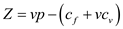….. (1) Where, - Z = the total profit or loss for a specified volume of units, - v = the amount of volume that is examined in the equation, - p = the price of one unit that is being measured here, - c f = the sum of fixed costs for the units produced, and - c v = the sum of variable costs for the units produced. Though this looks like a daunting problem and yucky formula, it really isn't all that hard once one knows what is going on. From the problem's text, we know that the fixed costs are \$8,000.00 and variable costs are approximately \$65.00 per table while the revenues generated per table are \$180.00. With the assumption that we have 300 tables produced and sold in an average month, all it takes is plugging in the numbers and seeing what we would end up with as a profit or loss value. These calculations are shown below for one to see: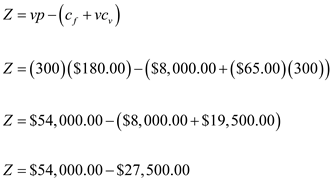Z = \$26,500.00 As of a result, the net profit from the production volume, revenues that are projected for each table and fixed and variable cost assumptions, the company would net a profit of \$26,500.00 based on 300 units sold. We can now turn ourselves to the second question which is another critical element to the concept of management science. (b) The second part of the problem that the author wants one to look at has to do with finding the monthly break even volume required in order for the company to meet revenues with equal expenses. Remember in this situation, the goal here is to have any net profit or loss equal to zero. Thus, the formula is a little bit different than what is was above and is shown below to continue along with the problem at hand: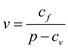….. (2) Where, - v = the amount of volume that is examined in the equation, - p = the price of one unit that is being measured here, - c f = the sum of fixed costs for the units produced, and - c v = the sum of variable costs for the units produced. Since we already know the values from the previous part of the problem above, it is a simple fractional equation that has to be solved here. The calculations are shown below for one to see as well as the answer: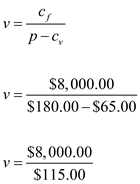v = 69.56 tables In order to achieve a break even status, the furniture company would need to produce 69.56 tables in order for any revenues to meet expenses. Anything that is above and beyond that is pure profit for the company. Note that one could say that they would need to produce 70 tables since it would be virtually impossible to produce a little more than half a table. Think of what that would look like…it would certainly not be a finished produce and probably fall to the ground the moment one puts something on it.